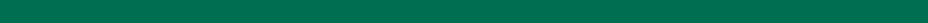>  >
Road Maps: A Guide to Learning System Dynamics

Road Maps 2: A Guide to Learning System Dynamics (D-4502-9)
This guide will lead you through the readings. The guide provides a summary of the important insights and highlights the system principles learned in each chapter of Road Maps.

The First Step (Leslie Martin) (D-4694)
A hands-on introduction to system dynamics modeling that uses a series of simple population models.

Beginner Modeling Exercises (Leslie Martin) (D-4347-7)
Exercises modeling constant flows. Develops understanding of the basic stock-and-flow structure through examples taken from a wide variety of systems.

An Introduction to Feedback (Leslie Martin) (D-4691)
A first look at the positive and negative feedback loops found in system dynamics models.

Graphical Integration Exercises Part1: Exogenous Rates  (A. Oh) (D-4547-1)
An introduction to graphical integration of constant flows and step function flows.

Introduction to Computer Simulation, Chapter 13 (N. Roberts et al.)
A simple and understandable explanation of levels and rates.

Formulating Models of Simple Systems Using Vensim PLE (Nelson Repenning) (D-4697-4)
A beginner's tutorial for Vensim PLE.